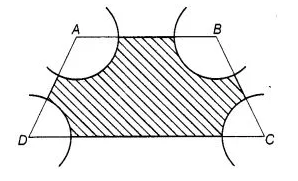# In figure, ABCD is a trapezium with AB || DC.

Question:

In figure, ABCD is a trapezium with AB || DC. AB = 18 cm, DC = 32 cm and distance between AB and DC = 14 cm. If arcs of equal radii 7 cm with

centres A, B, C and D have been drawn, then find the area of the shaded region of the figure.Solution:

Given, AB = 18 cm, DC = 32 cm, height, (h) = 14cm

and arc of radii $=7 \mathrm{~cm}$

Since, $A B \| D C$

$\therefore \quad \angle A+\angle D=180^{\circ}$

and $\angle B+\angle C=180^{\circ}$

$\therefore \quad$ Area of sector with angle $A$ and $D=\frac{\theta \times \pi r^{2}}{360}$

$=\frac{180^{\circ}}{360} \times \frac{22}{7} \times(7)^{2}$

$=11 \times 7=77 \mathrm{~cm}^{2}$

Similarly, area of sector with angle $B$ and $C=77 \mathrm{~cm}$

Now, $\quad$ area of trapezium $=\frac{1}{2}(A B+D C) \times h$

$=\frac{1}{2}(18+32) \times 14=\frac{50}{2} \times 14=350 \mathrm{~cm}^{2}$

$\therefore$ Area of shaded region $=$ Area of trapezium $-($ Area of sector points $A$ and $D$ + Area of sector points $B$ and $C$ )

$=350-(77+77)=196 \mathrm{~cm}^{2}$

Hence, the required area of shaded region is $1996 \mathrm{~cm}^{2}$